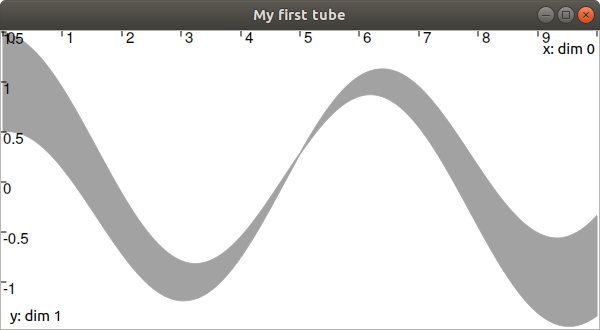# Start a MATLAB project¶

Tip

You are using Python?

Warning

MATLAB support is preliminary, limited tests have been made for now. See this example.
Codac is ready to be used if you have at least MATLAB R2019b, a supported version of Python and installed Codac Python package.

MATLAB specificities

Integers in function calls from the Codac library should be cast to int32, e.g. 4 would be int32(4) (however do not change those that are in strings), otherwise remember that all numbers are double by default in MATLAB.
The operator [index] for a Codac object should be replaced with .getitem(int32(index)) when getting its value while it should be replaced with .setitem(int32(index)) when setting its value (please note that indices of Codac objects start at 0 contrary to typical MATLAB objects such as MATLAB arrays or cell arrays).
The operators/functions x&y (intersection) for a Codac object should be replaced with x.inter(y), x|y (union) with x.union(y), x&=y (intersection and update of x) with x.inter_self(y), x|=y (union and update of x) with x.union_self(y), x**y (power) with x.pow(y), abs(x) (absolute) with x.abs().
Python lists of objects should be converted to MATLAB cell arrays.
Also, when a Codac function needs a py.list or Vector parameter, the corresponding MATLAB cell array should be given as py.list(…) (however when the Codac function do not need a py.list or Vector parameter but just an element of a MATLAB cell array, do not convert with py.list(…) and be sure to get the cell array element with {} operator).
Be sure that Python multiline strings are correctly converted to multiline MATLAB strings between . Remember that multiline statements in MATLAB need before next line.
Please also convert Python for loops to typical MATLAB for loops, same for if-else, += statements.

Automating Python to MATLAB conversions

Bing Chat with GPT-4 in Precise conversation style has been used successfully to help convert Python examples to MATLAB, using the description above and by providing 01_getting_started.py and the corresponding a01_getting_started.m as example (about 80% of the code for 03_static_rangebearing.m and 05_dyn_rangebearing.m was correctly converted this way).
import py.codac.*

x = Tube(Interval(0,10), 0.01, TFunction("cos(t)+abs(t-5)*[-0.1,0.1]"));

beginDrawing();
fig = VIBesFigTube("My first tube");